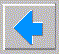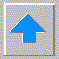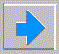# Lecture 5 - Search Methods

• Let's continue with search problems
• Blind search - no objective, just keep trying (RN s3.5)
• Search Example (RN p62) Romanian Travel
• depth first without recurrence check
• depth first with full recurrence check and backtracking
• depth limited
• iteratively increasing depth
• Informed search (RN ch4)
• we have a path cost
• assumed that only know true cost after expansion
• heuristics
• from Greek word for discovery
• h(n) such that h(n)=0 iff goal, h smaller for better nodes
• examples - straight line distance, 8-puzzle
• how to find heuristic ?
• optimistic heuristics (less costly than actual)
• always underestimate cost
• triangle inequality heuristics
• best first search
• minimal accumulative cost on fringe g(n) (complete, optimal, but slow)
• special case - breadth first
• optimal if monotonic increasing cost (eg. additive)
• greedy heuristics (neither complete nor optimal, but often fast)
• A* (RN pp96-101)
• combination of best of above two f(n) = g(n) + h(n)
• optimal for optimistic heuristics
• optimally efficient - no other optimal algorithm always expands fewer nodes
• but still exponential for additive costs
• why does it work? equicost contours
• Examples - Romanian cities, 8-puzzle (cost >= h2 >= h1)
• A* variants
• Philosophical restrictions of search techniques
• search wasteful since doesn’t reason
• what's missing ?
• there is a mathematically developed theory of logic - basis of all math!